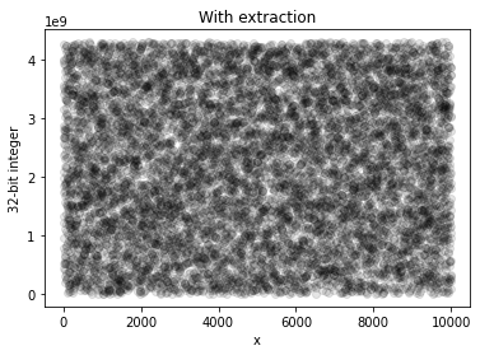# Qiskit RNG (0.2.2)¶

Caution

This package is currently provided in beta form and heavy modifications to both functionality and API are likely to occur.Qiskit RNG is a collaboration between Cambridge Quantum Computing (CQC) and IBM Quantum for generating certified quantum randomness using IBM Quantum systems running three-qubit GHZ states with random X- and Y-measurements. Provided that the state is tripartite entangled, as measurement by a violation of the Mermin inequality ($$x,y\in \{1,2\}$$):

$M_{\rm obs} = \langle A_{1}B_{1}C_{1}\rangle - \langle A_{1}B_{2}C_{2}\rangle - \langle A_{2}B_{1}C_{2}\rangle - \langle A_{2}B_{2}C_{1}\rangle \le 2,$

the measured output can be certified quantum random for values $$2\sqrt{2} \le M_{\rm obs} \le 4$$; the underlying state was truly tripartite entangled. The maximum value $$M_{\rm obs} = 4$$ indicates maximum randomness, and and that the measurement outcomes are uniformly random. These “raw bits” are passed through a two-source randomness extractor that creates a reduced length uniform random distribution (if $$M_{\rm obs} \neq 4$$) of bits.

Further technical details maybe found in: Foreman et al., “Practical randomness and privacy amplification”, arXiv:2009.06551.

qiskit_rng has passed the following RNG test suites:

## Quick start¶

from qiskit import IBMQ
from qiskit_rng import Generator